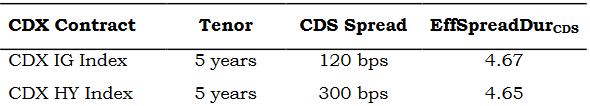# Offsetting CDS positions

Synthetic Credit Strategies: Economic Slowdown Scenario
As in the prior example, an active fixed-income manager anticipates an economic slowdown in the next year with a greater adverse impact on lower- rated issuers. The manager chooses a tactical CDX (credit default swap index) strategy combining positions in investment-grade and high-yield CDX contracts to capitalize on this view. The current market information for investment-grade and high-yield CDX contracts is as follows:Assume that both CDX contracts have a \$10,000,000 notional with premiums paid annually, and that the EffSpreadDurCDS for the CDX IG and CDX HY contracts in one year are 3.78 and 3.76, respectively. We ignore the time value of money for purposes of this example.

1. Describe the appropriate tactical CDX strategy and calculate the one-year return assuming no change in credit spread levels.
2. Calculate the one-year return on the tactical CDX strategy under an economic downturn scenario in which investment-grade credit spreads rise by 50% and high-yield credit spreads double.
CDS Price ≈ 1 + ((Fixed Coupon – CDS Spread) ⨯ EffSpreadDurCDS/100)

Solution to 1:
The investor should initially buy protection on the CDX HY Index and sell protection on the CDX IG Index. Current CDS prices are estimated by multiplying EffSpreadDurCDS by the spread difference from the standard rates of 1% and 5%, respectively:
CDX HY: 109.3 per \$100 face value, or 1.093 (= 1 + (5.00% – 3.00% × 4.65/100))
CDX IG: 99.066 per \$100 face value, or 0.99066 (= 1 + (1.00% – 1.20% × 4.67/100))

Since the investor is both buying HY protection at a premium to par (that is, agreeing to pay the 5% standard coupon while the underlying CDS spread is 3.00%) and selling IG protection at a discount from par (or agreeing to receive the standard 1.00% while the underlying index spread is 1.20%), the investor will receive an upfront payment for entering both positions as follows:
\$1,023,400 = [\$10,000,000 × (1.093 – 1)] + [\$10,000,000 × (1 – 0.99066)]

In one year, the return is measured by combining the net CDX coupon income or expense with the price appreciation assuming no spread change. As the investor is long CDX HY protection (i.e., pays the 5.00%
standard HY coupon) and short CDX IG protection (or receives the standard 1.00% IG coupon), the net annual premium paid by the investor at year end is \$400,000 (=\$10,000,000 × (5.00% - 1.00%). The respective CDS prices in one year are as follows:
CDX HY: 107.52 per \$100 face value, or 1.0752 (=1 + (2.00% × 3.76))
CDX IG: 99.244 per \$100 face value, or 0.99244 (=1 + (−0.20% × 3.78))

To offset the existing CDX positions in one year, the investor would sell HY protection and buy IG protection. The investor is able to sell HY protection at a premium of 7.52, resulting in a \$178,000 gain from the long CDX HY position over one year (1.093 – 1.0752) × \$10,000,000).

Since the investor must buy IG protection in one year at a lower discount to par of (1 – 0.99244), it has a \$17,800 gain from the CDX IG position (= (0.99244 – 0.99066) × \$10,000,000). Subtracting the \$400,000 net coupon payment made by the investor results in a one-year loss from the strategy of \$204,200 (= \$178,000 + \$17,800 – \$400,000) with constant spreads.

Solution to 2:
Initial CDS prices are derived exactly as in Question 1:
CDX HY: 109.3 per \$100 face value, or 1.093 (= 1 + (2.00% × 4.65))
CDX IG: 99.066 per \$100 face value, or 0.99066 (= 1 + (–0.2% × 4.67))

In one year, the return is measured by combining the coupon income with the price appreciation given the rise in the CDX IG spread to 1.80% (increased by 60 bps) and the CDX HY spread to 6.00% (increased by 300 bps). In this case, the investor takes the same position to that of Question 1— namely, long CDX HY protection (short risk) and short CDX IG protection (long risk), so the net annual premium paid remains \$400,000 (=\$10,000,000 × (5.00% – 1.00%). Respective CDS prices in one year are as follows:
CDX HY: 96.24 per \$100 face value, or 0.9624 (=1 + (–1.00% × 3.76))
CDX IG: 96.976 per \$100 face value, or 0.96976 (=1 + (–0.80% × 3.78)

When offsetting the transaction in one year, the investor suffers a \$209,000 loss from the short CDX IG position ((0.99066 – 0.96976) × –\$10,000,000) and benefits from a \$1,306,000 gain from offsetting the
CDX HY position (1.093 – 0.9624) × \$10,000,000). Subtracting the \$400,000 net premium paid results in a one-year gain from the strategy of \$697,000 (= \$1,306,000 – \$209,000 - \$400,000) under the second scenario.

Under Question 2, when offsetting positions in one year, meaning, buying protection on IG, selling protection on HY, isn’t it so that he now buys protection at a discount, so it is a gain; and sells protection on HY, at a discount, leading to a loss?

I am struggling too with this problem, especially with the offsetting positions. As for solution 1, I felt they were both a loss given that for HY, the price went down and for IG, the price went high

1 Like

If you are buying CDS HY, you gain when the price drops because CDS spread is rising here and if are selling the CDS IG, you will lose when price rises because CDS spread is also widening here.

Easy way to solve the question is figure out which side of the trade you are on, see if the spread has narrowed or widened and then solve the question, everything falls in place

To be clear: when spreads widen, CDS prices increase; when spreads narrow, CDS prices decrease.

Buying a CDS is akin to shorting a bond.